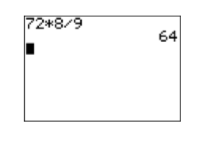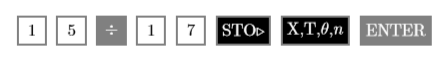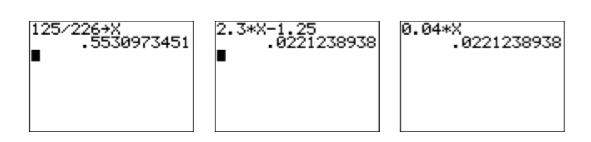$$\newcommand{\id}{\mathrm{id}}$$ $$\newcommand{\Span}{\mathrm{span}}$$ $$\newcommand{\kernel}{\mathrm{null}\,}$$ $$\newcommand{\range}{\mathrm{range}\,}$$ $$\newcommand{\RealPart}{\mathrm{Re}}$$ $$\newcommand{\ImaginaryPart}{\mathrm{Im}}$$ $$\newcommand{\Argument}{\mathrm{Arg}}$$ $$\newcommand{\norm}{\| #1 \|}$$ $$\newcommand{\inner}{\langle #1, #2 \rangle}$$ $$\newcommand{\Span}{\mathrm{span}}$$

# 2.3: Clearing Fractions and Decimals

•• Contributed by David Arnold
• Retired Professor (Mathematics) at College of the Redwoods
$$\newcommand{\vecs}{\overset { \rightharpoonup} {\mathbf{#1}} }$$ $$\newcommand{\vecd}{\overset{-\!-\!\rightharpoonup}{\vphantom{a}\smash {#1}}}$$$$\newcommand{\id}{\mathrm{id}}$$ $$\newcommand{\Span}{\mathrm{span}}$$ $$\newcommand{\kernel}{\mathrm{null}\,}$$ $$\newcommand{\range}{\mathrm{range}\,}$$ $$\newcommand{\RealPart}{\mathrm{Re}}$$ $$\newcommand{\ImaginaryPart}{\mathrm{Im}}$$ $$\newcommand{\Argument}{\mathrm{Arg}}$$ $$\newcommand{\norm}{\| #1 \|}$$ $$\newcommand{\inner}{\langle #1, #2 \rangle}$$ $$\newcommand{\Span}{\mathrm{span}}$$ $$\newcommand{\id}{\mathrm{id}}$$ $$\newcommand{\Span}{\mathrm{span}}$$ $$\newcommand{\kernel}{\mathrm{null}\,}$$ $$\newcommand{\range}{\mathrm{range}\,}$$ $$\newcommand{\RealPart}{\mathrm{Re}}$$ $$\newcommand{\ImaginaryPart}{\mathrm{Im}}$$ $$\newcommand{\Argument}{\mathrm{Arg}}$$ $$\newcommand{\norm}{\| #1 \|}$$ $$\newcommand{\inner}{\langle #1, #2 \rangle}$$ $$\newcommand{\Span}{\mathrm{span}}$$

In this section we introduce techniques that clear fractions and decimals from equations, making the resulting equation a lot easier to solve. When clearing fractions from an equation, you will need to simplify products like the ones posed in the following examples.

Example $$\PageIndex{1}$$

Simplify: $$12\left(\dfrac{2}{3} x\right)$$.

Solution

When we multiply three numbers, such as $$12$$, $$2/3$$, and $$x$$, the associative property of multiplication tells us that it does not matter which two numbers we multiply ﬁrst. We use the associative property to regroup, then multiply numerators and denominators and simplify the result.

\begin{aligned} 12\left(\dfrac{2}{3} x\right) &=\left(12 \cdot \dfrac{2}{3}\right) x \quad \color{Red} \text{Associative property of multiplication.}\\ &=\dfrac{24}{3} x \quad \color{Red} \text { Multiply: } 12 \cdot 2=24 \\ &=8 x \quad \color{Red} \text { Divide: } 24 / 3=8 \end{aligned} \nonumber

Exercise $$\PageIndex{1}$$

Simplify: $$15\left(\dfrac{3}{5} x\right)$$.

$$9x$$

Example $$\PageIndex{1}$$ shows all of the steps involved in arriving at the answer. However, the goal in this section is to perform this calculation mentally. So we just “Multiply $$12$$ and $$2$$ to get $$24$$, then divide $$24$$ by $$3$$ to get $$8$$.” This approach allows us to write down the answer without doing any work.

$12\left(\dfrac{2}{3} x\right)=8 x \nonumber$

You should practice this mental calculation until you can write down the answer without writing down any steps.

Example $$\PageIndex{2}$$

Simplify: $$18 \left(\dfrac{2}{9} x\right)$$.

Solution

This time we perform the calculations mentally. Multiply $$18$$ and $$2$$ to get $$36$$, then divide $$36$$ by $$9$$ to get $$4$$.

$18\left(\dfrac{2}{9} x\right)=4 x \nonumber$

Exercise $$\PageIndex{2}$$

Simplify: $$14 \left(\dfrac{3}{7} x\right)$$.

$$6x$$

When the numbers get larger, the mental calculations become harder. For example, consider $72\left(\dfrac{8}{9} x\right) \nonumber$

In this case, the work “multiply $$72$$ and $$8$$ to get $$576$$, then divide $$576$$ by $$9$$ to get $$64$$” is a bit diﬃcult to carry in your head. However, this is when the calculator comes to the rescue.

Example $$\PageIndex{3}$$

Use your calculator to help simplify $$72 \left(\dfrac{8}{9} x\right)$$.

Solution

Use your calculator to multiply $$72$$ and $$8$$, then divide by $$9$$. Enter 72*8/9 and press the ENTER key.Thus, $$72\left(\dfrac{8}{9} x\right)=64 x$$.

Exercise $$\PageIndex{3}$$

Use your calculator to simplify: $$81 \left(\frac{5}{9} x\right)$$.

$$45x$$

### Canceling is more eﬃcient

In Examples $$\PageIndex{1}$$, $$\PageIndex{2}$$, and $$\PageIndex{3}$$, we multiplied numerators, then divided by the sole denominator. We also saw that it is a bit diﬃcult to carry the work in our head as the numbers grow larger. In Chapter 1, Section 3, we saw that canceling reduces the size of the numbers and simpliﬁes the work.

Example $$\PageIndex{4}$$

Simplify: $$72 \left(\dfrac{8}{9} x\right)$$.

Solution

In Example $$\PageIndex{3}$$, we used our calculator to multiply $$72$$ and $$8$$ to get $$576$$, then divided $$576$$ by $$9$$ to get $$64$$. In this solution, we divide $$9$$ into $$72$$ to get $$8$$, then multiply $$8$$ by $$8$$ to get $$64$$. We get the same answer, but because the intermediate numbers are much smaller, the calculations are much easier to do mentally.

\begin{aligned} 72\left(\dfrac{8}{9} x\right) &=\left(72 \cdot \dfrac{8}{9}\right) x \quad \color{Red} \text{Associative property of multiplication}\\ &=(8 \cdot 8) x \quad \color{Red} \text { Divide: } 72 / 9=8 \\ &=64 x \quad \color{Red} \text { Multiply: } 8 \cdot 8=64 \end{aligned} \nonumber

Exercise $$\PageIndex{4}$$

Simplify: $$64 \left(\dfrac{5}{8} x\right)$$.

$$40x$$

Example $$\PageIndex{4}$$ shows all of the steps involved in arriving at the answer. Again, the goal in this section is to perform this calculation mentally, so we just “Divide $$9$$ into $$72$$ to get $$8$$, then multiply $$8$$ by $$8$$ to get $$644$$.”

$72\left(\frac{8}{9} x\right)=64 x \nonumber$

Not only does this approach allow us to write down the answer without doing any work, the numerical calculations involve smaller numbers. You should practice this mental calculation until you can write down the answer without writing down any steps.

Example $$\PageIndex{5}$$

Simplify: $$27\left(\dfrac{5}{9} x\right)$$.

Solution

Divide $$9$$ into $$27$$ to get $$3$$, then multiply $$3$$ by $$5$$ to get $$15$$. $27\left(\dfrac{5}{9} x\right)=15 x \nonumber$

Exercise $$\PageIndex{5}$$

Simplify: $$18\left(\dfrac{3}{2} x\right)$$.

$$27x$$

Note

The technique shown in Examples $$\PageIndex{4}$$ and $$\PageIndex{5}$$ is the technique we’ll use in the remainder of this section. Dividing (canceling) ﬁrst is far more eﬃcient, the smaller numbers allowing us to perform the calculation mentally.

### Clearing Fractions from an Equation

Now that we’ve done the required fraction work, we can now concentrate on clearing fractions from an equation. Once the fractions are removed from the equation, the resulting equivalent equation is far easier to solve than the original.

Clearing fractions from an equation

To clear fractions from an equation, multiply both sides of the equation by the least common denominator.

Example $$\PageIndex{6}$$

Solve for $$x : \quad x+\dfrac{2}{3}=\dfrac{1}{2}$$.

Solution

The common denominator for $$2/3$$ and $$1/2$$ is $$6$$. We begin by multiplying both sides of the equation by $$6$$.

\begin{aligned} x+\dfrac{2}{3}&= \dfrac{1}{2} \quad \color{Red} \text { Original equation. } \\ 6\left(x+\dfrac{2}{3}\right) &= 6\left(\dfrac{1}{2}\right) \quad \color{Red} \text{ Multiply both sides by 6. } \\ 6x+6\left(\dfrac{2}{3}\right)&= 6\left(\dfrac{1}{2}\right)\quad \color{Red} \text { On the left, distribute the } 6 \end{aligned} \nonumber

To simplify $$6(2/3)$$, divide $$6$$ by $$3$$ to get $$2$$, then multiply $$2$$ by $$2$$ to get $$4$$. Thus, $$6(2/3) = 4$$. Similarly, $$6(1/2) = 3$$.

$6x+4=3 \quad \color{Red} \text {Multiply: } 6\left(\dfrac{2}{3}\right)=4,6\left(\dfrac{1}{2}\right)=3 \nonumber$

Note that the fractions are now cleared from the equation. To isolate terms containing $$x$$ on one side of the equation, subtract $$4$$ from both sides of the equation.

\begin{aligned} 6x+4-4&= 3-4 \quad \color{Red} \text { Subtract } 4 \text { from both sides. } \\ 6x&= -1 \quad \color{Red} \text { Simplify both sides. } \end{aligned} \nonumber

To “undo” multiplying by $$6$$, divide both sides by $$6$$.

\begin{aligned} \dfrac{6x}{6}&= \dfrac{-1}{6} \quad \color{Red} \text { Divide both sides by } 6 \\ x&= -\dfrac{1}{6} \quad \color{Red} \text { Simplify both sides. } \end{aligned} \nonumber

Check: Let’s use the TI-84 to check the solution.

1. Store $$-1/6$$ in the variableX using the following keystrokes.Figure $$\PageIndex{1}$$.
1. Enter the left-hand side of the original equation: $$x +2 /3$$. Use the following keystrokes.Figure $$\PageIndex{1}$$.
1. Press the MATH key, then select 1:►Frac and press the ENTER key. The result is shown in the third line in Figure $$\PageIndex{1}$$.Figure $$\PageIndex{1}$$: Checking that $$-1/6$$ is a solution of $$x +2/3=1 /2$$.

The result is identical to the right-hand side of the equation $$x +2 /3=1 /2$$. Thus, the solution checks.

Exercise $$\PageIndex{6}$$

Solve for $$x : x-\dfrac{3}{4}=\dfrac{1}{2}$$.

$$x=5 / 4$$

Example $$\PageIndex{7}$$

Solve for $$x : \quad \dfrac{4}{5} x=-\dfrac{4}{3}$$.

Solution

The common denominator for $$4/5$$ and $$-4/3$$ is $$15$$. We begin by multiplying both sides of the equation by $$15$$.

\begin{aligned} \dfrac{4}{5} x& =-\dfrac{4}{3} \quad \color{Red} \text { Original equation. } \\ 15\left(\dfrac{4}{5} x\right) & =15\left(-\dfrac{4}{3}\right) \quad \color{Red} \text { Multiply both sides by } 15 \end{aligned} \nonumber

To simplify $$15(4/5)$$, divide $$5$$ into $$15$$ to get $$3$$, then multiply $$3$$ by $$4$$ to get $$12$$. Thus, $$15(4/5) = 12$$. Similarly, $$15(-4/3) = -20$$

$12x=-20 \quad \color{Red} \text{Multiply.} \nonumber$

To “undo” multiplying by $$12$$, we divide both sides by $$12$$.

\begin{aligned} \dfrac{12x}{12}& =\dfrac{-20}{12} \quad \color{Red} \text { Divide both sides by } 12 \\ x & =-\dfrac{5}{3} \quad \color{Red} \text { Reduce to lowest terms. } \end{aligned} \nonumber

Check: To check, substitute $$-5/3$$ for $$x$$ in the original equation.

\begin{aligned} \dfrac{4}{5} x &=-\dfrac{4}{3} \quad \color{Red} \text { Original equation. }\\ \dfrac{4}{5}\left(-\dfrac{5}{3}\right) &=-\dfrac{4}{3} \quad \color{Red} \text { Substitute } -5 / 3 \text {for} x \\ -\dfrac{20}{15} &=-\dfrac{4}{3} \quad \color{Red} \text { Multiply numerators and denominators. }\\ -\dfrac{4}{3} &=-\dfrac{4}{3} \quad \color{Red} \text { Reduce. } \end{aligned} \nonumber

The fact that the last line is a true statement guarantees the $$-5/3$$ is a solution of the equation $$\dfrac{4}{5} x=-\dfrac{4}{3}$$

Exercise $$\PageIndex{7}$$

Solve for $$x : -\dfrac{3}{7} x=\dfrac{3}{2}$$.

$$x=-7 / 2$$

Example $$\PageIndex{8}$$

Solve for $$x : \dfrac{2 x}{3}-\dfrac{3}{4}=\dfrac{1}{2}-\dfrac{3 x}{4}$$.

Solution

The common denominator for $$2x/3$$, $$-3/4$$, $$1/2$$, and $$-3x/4$$ is $$12$$. We begin by multiplying both sides of the equation by $$12$$.

\begin{aligned} \dfrac{2 x}{3}-\dfrac{3}{4}&= \dfrac{1}{2}-\dfrac{3 x}{4} \quad \color{Red} \text { Original equation. } \\ 12\left(\dfrac{2x}{3}-\dfrac{3}{4}\right)&= 12\left(\dfrac{1}{2}-\dfrac{3x}{4}\right) \quad \color{Red} \text { Multiply both sides by } 12 \\ 12\left(\dfrac{2x}{3}\right)-12\left(\dfrac{3}{4}\right)&= 12\left(\dfrac{1}{2}\right)-12\left(\dfrac{3x}{4}\right) \quad \color{Red} \text { Distribute the } 12 \text { on each side. } \end{aligned} \nonumber

To simplify $$12(2x/3)$$, divide $$3$$ into $$12$$ to get $$4$$, then multiply $$4$$ by $$2x$$ to get $$8x$$. Thus, $$12(2x/3) = 8x$$. Similarly, $$12(3/4) = 9$$, $$12(1/2) = 6$$, and $$12(3x/4) = 9x$$.

$8x-9=6-9x \quad \color{Red} \text {Multiply.} \nonumber$

Note that the fractions are now cleared from the equation. We now need to isolate terms containing $$x$$ on one side of the equation. To remove the term $$-9x$$ from the right-hand side, add $$9x$$ to both sides of the equation.

\begin{aligned} 8x-9+9x&= 6-9x+9x \quad \color{Red} \text { Add } 9x \text { to both sides. } \\ 17x-9&= 6 \quad \color{Red} \text { Simplify both sides. } \end{aligned} \nonumber

To remove the term $$-9$$ from the left-hand side, add $$9$$ to both sides of the equation.

\begin{aligned} 7x-9+9&= 6+9 \quad \color{Red} \text { Add } 9 \text { to both sides. } \\ 17x&= 15 \quad \color{Red} \text { Simplify both sides. } \end{aligned} \nonumber

Finally, to “undo” multiplying by $$17$$, divide both sides of the equation by $$17$$.

\begin{aligned} \dfrac{17x}{17} &= \dfrac{15}{17} \quad \color{Red} \text { Divide both sides by } 17 \\ x &= \dfrac{15}{17} \quad \color{Red} \text { Simplify both sides. } \end{aligned} \nonumber

Check: Let’s use the TI-84 to check the solution.

1. Store the number $$15/17$$ in the variable $$X$$ using the following keystrokes.Figure $$\PageIndex{2}$$.Figure $$\PageIndex{2}$$: Storing $$15/17$$ in $$X$$.
1. Enter the left-hand side of the original equation: $$\dfrac{2 x}{3}-\dfrac{3}{4}$$. Use the following keystrokes.Figure $$\PageIndex{3}$$.
1. Enter the right-hand side of the original equation: $$\dfrac{1}{2}-\dfrac{3 x}{4}$$. Use the following keystrokes.Figure $$\PageIndex{3}$$.

Because both sides simplify to $$-.1617647059$$ when $$15/17$$ is substituted for $$x$$, this guarantees that $$15/17$$ is a solution of the equation $$2x/3-3/4=1/2-3x/4$$.Figure $$\PageIndex{3}$$: Checking $$15/17$$ in $$2x/3-3/4=1 /2-3x/4$$.

Exercise $$\PageIndex{8}$$

Solve for $$x : \dfrac{5 x}{9}-\dfrac{2}{3}=\dfrac{5}{9}-\dfrac{3 x}{2}$$.

$$x=22 / 37$$

### Clearing Decimals from an Equation

Multiplying by the appropriate power of ten will clear the decimals from an equation, making the resulting equivalent equation far easier to solve.

Before we begin, ﬁrst recall the following facts about multiplying by powers of ten.

• $$10(1.2345) = 12.345$$. Multiplying by $$10$$ moves the decimal point one place to the right.
• $$100(1.2345) = 123.45$$. Multiplying by $$100$$ moves the decimal point two places to the right.
• $$1000(1.2345) = 1234.5$$. Multiplying by $$1000$$ moves the decimal point three places to the right.

Note the pattern: The number of zeros in the power of ten determines the number of places to move the decimal point. So, for example, if we multiply by $$1,000,000$$, which has six zeros, this will move the decimal point six places to the right.

Example $$\PageIndex{9}$$

Solve for $$x : \quad 2.3 x-1.25=0.04 x$$.

Solution

The ﬁrst term of $$2.3x-1.25 = 0.04x$$ has one decimal place, the second term has two decimal places, and the third and ﬁnal term has two decimal places. At a minimum, we need to move each decimal point two places to the right in order to clear the decimals from the equation. Consequently, we multiply both sides of the equation by $$100$$.

\begin{aligned} 2.3 x-1.25&= 0.04 x \quad \color{Red} \text { Original equation. } \\ 100(2.3 x-1.25)&= 100(0.04 x) \quad \color{Red} \text { Multiply both sides by } 100 \\ 100(2.3 x)-100(1.25)&= 100(0.04 x) \quad \color{Red} \text { Distribute the } 100 \text { . } \\ 230 x-125&= 4 x \quad \color{Red} \text { Multiplying by } 100 \text { moves all decimal points two places to the right. } \end{aligned} \nonumber

Note that the decimals are now cleared from the equation. We must now isolate all terms containing x on one side of the equation. To remove the term $$4x$$ from the right-hand side, subtract $$4x$$ from both sides of the equation.

\begin{aligned} 230x-125-4x&= 4x-4x \quad \color{Red} \text { Subtract } 4x \text { from both sides. } \\ 226x-125&=0 \quad \color{Red} \text { Simplify. } \end{aligned} \nonumber

To remove $$-125$$ from the left-hand side, add $$125$$ to both sides of the equation.

\begin{aligned} 226x-125+125&= 0+125 \quad \color{Red} \text { Add } 125 \text { to both sides. } \\ 226x&= 125 \quad \color{Red} \text { Simplify both sides. } \end{aligned} \nonumber

Finally, to “undo” multiplying by $$226$$, divide both sides by $$226$$.

\begin{aligned} \dfrac{226x}{226} &= \dfrac{125}{226} \quad \color{Red} \text { Divide both sides by } 226 \\ x &= \dfrac{125}{226} \quad \color{Red} \text { Simplify. } \end{aligned} \nonumber

Check: Let’s check the answer with the TI-84.

1. Store $$125/226$$ in the variable $$X$$ using the following keystrokes.The result is shown in the ﬁrst image in $$\PageIndex{4}$$.

1. Enter the left-hand side of the original equation: $$2.3x-1.25$$. Use the following keystrokes.Figure $$\PageIndex{4}$$.
1. Enter the right-hand side of the original equation: 0.04x. Use the following keystrokes.Figure $$\PageIndex{4}$$.Figure $$\PageIndex{4}$$: Checking $$125/226$$ in $$2.3x-1.25 = 0.04x$$.

Note that both sides yield the same decimal approximation $$0.0221238938$$ when $$125/226$$ is substituted for $$x$$. This guarantees that $$125/226$$ is a solution of $$2.3x-1.25 = 0.04x$$.

Exercise $$\PageIndex{9}$$

Solve for $$x : 1.34-4.5 x=2.2$$.

$$x=-43 / 225$$